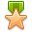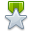×

# Am I missing something here? Is the right answer here? If so can someone explain?

######Jump to latest post
Page 2 of 2First 12
1.Member
Join Date
Jun 2021
Location
Houston,TX
Posts
26
Reputation## That's what I thought

I was trying it the way you described because that's what I had been taught. And like we have figured out it is not an answer choice. And yes it was from the pool of questions in my NETA 2 Practice Test. Unfortunately I think the question is ignoring the 4-20mA and treating it like a 0-20mA because of the answer choices available.Originally Posted by Kalbi_RobI think ya'll have a slight conceptual misunderstanding of how transducers work. Transducer typically output range of 4mA to 20mA. That means 4mA equals the low range minimum, and 20mA is high range maximum. For a 0-150A CT, 4mA is equal to 0A and 20mA is equal to 150A.

So, I've always just made my range 0mA to 16mA, with my reading minus 4.

So, at 14mA, my calculation would be (10mA*150A)/16mA = 93.75, so my guess would be the answer of 90A due the possibility of inaccurate.

Not sure if this question is the best example to work off of, did you get this from the question bank? Might be one to bring up to the administrators.

To check my work I use calculators similar to this one:

https://www.sensorsone.com/4-20ma-to...&olo=0&ohi=150Reply With Quote

2.Member
Join Date
Jul 2020
Location
Washington, United State
Posts
44
ReputationOriginally Posted by cnowakI was trying it the way you described because that's what I had been taught. And like we have figured out it is not an answer choice. And yes it was from the pool of questions in my NETA 2 Practice Test. Unfortunately I think the question is ignoring the 4-20mA and treating it like a 0-20mA because of the answer choices available.Reply With Quote

3.NETA Level III Pro Subscriber
Join Date
Dec 2019
Location
Richmond VA
Posts
51
ReputationSooo testguy says 105 is right, are we voting this one wrong?Reply With Quote

4.Originally Posted by bec51392Sooo testguy says 105 is right, are we voting this one wrong?
Thanks for reporting, this question will be updated. See answer explination by Kalbi_Rob: https://testguy.net/threads/8410-Am-...4735#post14735Reply With Quote

5.NETA Level III Pro Subscriber
Join Date
Dec 2019
Location
Richmond VA
Posts
51
ReputationI took my NETA 3 this week and this question ended up on my test! Thanks to you guys I think its fair to say I got it right. Thanks everyone!Reply With Quote

6. ## CT ratioOriginally Posted by cnowakA 4-20mA single CT has a max range of 150 amp what is the amps at 14mA?

140A

105A

150A

90A

105A
150 divided by 20 is 7.5
That would be 7.5mA on secondary per 1 amp on primary.
at 14mA on secondary (14 x 7.5 = 105) that would be 105 amp on primary.
Ignore the 4 in the 4-20mA range as that is just a pickup for accuracy. Even using on 16 or 17 for the span of the range of the 4-20mA, the answer would be 123A or 131A, which is not an option, so the A per mA ratio is based on the 150 divided by 20 and ignores the lower limit of 4.Reply With Quote

7. ## low limitOriginally Posted by Kalbi_RobI think ya'll have a slight conceptual misunderstanding of how transducers work. Transducer typically output range of 4mA to 20mA. That means 4mA equals the low range minimum, and 20mA is high range maximum. For a 0-150A CT, 4mA is equal to 0A and 20mA is equal to 150A.

So, I've always just made my range 0mA to 16mA, with my reading minus 4.

So, at 14mA, my calculation would be (10mA*150A)/16mA = 93.75, so my guess would be the answer of 90A due the possibility of inaccurate.

Not sure if this question is the best example to work off of, did you get this from the question bank? Might be one to bring up to the administrators.

To check my work I use calculators similar to this one:

https://www.sensorsone.com/4-20ma-to...&olo=0&ohi=150

Using that calculator with 30 as the Low Limit, it calculates the primary current to be 105A.Reply With Quote

8.Junior Member Pro Subscriber
Join Date
Jun 2021
Posts
1
ReputationOriginally Posted by BruceG400Using that calculator with 30 as the Low Limit, it calculates the primary current to be 105A.

If this is a transducer, then at 0 amps the output will be 4mA.
So, rather than the lower limit being 30A by calculating 150A/20mA as the max outputs, we are instead calculating 150A/(20-4) to adjust for the 4mA offset. So it is instead 150/16=9.375mA per primary Amp
Then use this reading and multiply it by the corrected secondary reading ie (14mA-4mA)=10

9.375*10=93.75AReply With Quote

codes and standards, current transformers, electrical calculations, electrical theory, neta level 2